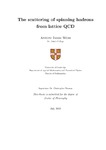## The scattering of spinning hadrons from lattice QCD

##### Authors
Woss, Antoni Jameshttps://orcid.org/0000-0002-7377-8812
##### DOIThesis
##### Abstract

Hadron spectroscopy is predominantly the study of resonances that decay via the strong interaction into a multitude of stable hadrons, such as the pion. The vast majority of resonances decay via an intermediate hadron with non-zero intrinsic spin. In this thesis, I will present the results of scattering calculations featuring mesons with non-zero intrinsic spin. Before doing so, I will first give a brief introduction to QCD and review the framework necessary to perform lattice QCD calculations in Chapters 1 and 2.

In Chapter 3, I present the first lattice calculation of ρπ scattering in isospin-2. Here, ρπ features in dynamically-coupled 3S1 and 3D1 partial-waves with JP=1+. No resonance enhancement is anticipated in the flavour exotic isospin-2 channel and as such it provides an ideal testing ground for this first calculation. I work at heavier than physical quark masses at the SU(3)F point where the up, down and strange quarks are mass degenerate. Finite-volume spectra are calculated and, utilising the relationship between the discrete energy spectrum and the infinite-volume scattering amplitudes, partial-wave amplitudes with J≤3 and the degree of dynamical mixing between the coupled 3S1 and 3D1 channels are determined.

In Chapter 4, I investigate ρπ in isospin-1 where the a1 axial-vector resonance is expected to feature. Here, I present a discussion on G-parity and Bose-symmetry at the SU(3)F point. Working at heavier than physical quark masses, the resulting finite volume spectrum suggests that the a1 is a bound-state and that the 3S1- and 3D1-wave, ρπ scattering amplitudes are similar to those in isospin-2.

I present the first calculation of coupled πω and πϕ scattering in Chapter 5 where resonant enhancement is seen experimentally in the JP=1+ channel. Working at a somewhat lighter pion mass than in previous chapters, the finite-volume spectra are determined and the scattering amplitudes are calculated. Analytically continuing the amplitudes into the complex energy plane, a resonance pole is found, interpreted as the analogue of the b1 axial-vector, which couples dominantly to 3S1-wave πω, with a much-suppressed coupling to 3D1-wave πω, and a negligible coupling to πϕ.

In Chapter 6, the exotic JPC=1−+ channel is studied. These quantum numbers are not allowed in the quark model but can be obtained, for example, through a gluonic excitation coupled to a quark-antiquark pair. In this exploratory calculation, performed at the SU(3)F point, the finite-volume spectra and coupled-channel scattering amplitudes are presented. A single resonance pole is found, interpreted as the exotic π1, and couplings to meson-meson channels, including for example πη{1P1}, πη′{1P1} and ρπ{3P1}, are calculated for the first time in lattice QCD.

In order to minimally present the contents of a unitary n-channel scattering matrix, I introduce, in Chapter 7, an n-channel generalisation of the traditional two-channel Stapp parameterisation.

2019-07-30